Updating search results...

# 42 Results

View
Selected filters:
• NC.M1.A-CED.1Conditional Remix & Share Permitted
CC BY-NC-SA
Rating
0.0 stars
Overview:

CK-12's Texas Instruments Algebra I Student Edition Flexbook allows students to better utilize a graphing calculator in understanding the fundamental concepts of algebra.

Subject:
Mathematics
Material Type:
Textbook
Provider:
CK-12 Foundation
Provider Set:
CK-12 FlexBook
Author:
Jordan, Lori
12/08/2010Conditional Remix & Share Permitted
CC BY-NC-SA
Rating
0.0 stars
Overview:

CK-12 Foundation's Algebra FlexBook is an introduction to algebraic concepts for the high school student. Topics include: Equations & Functions, Real Numbers, Equations of Lines, Solving Systems of Equations & Quadratic Equations.

Subject:
Mathematics
Material Type:
Textbook
Provider:
CK-12 Foundation
Provider Set:
CK-12 FlexBook
Author:
Gloag, Andrew
Gloag, Anne
11/23/2010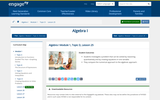Conditional Remix & Share Permitted
CC BY-NC-SA
Rating
0.0 stars
Overview:

Students investigate a problem that can be solved by reasoning quantitatively and by creating equations in one variable.
They compare the numerical approach to the algebraic approach.

Subject:
Math 1
Material Type:
Lesson
Author:
EngageNY
02/02/2020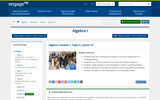Conditional Remix & Share Permitted
CC BY-NC-SA
Rating
0.0 stars
Overview:

Students learn the meaning and notation of recursive sequences in a modeling setting.
Students use recursive sequences to model and answer problems.
Students create equations and inequalities to solve a modeling problem.
Students represent constraints by equations and inequalities and interpret solutions as viable or non-viable options in a modeling context.

Subject:
Math 1
Material Type:
Lesson
Author:
EngageNY
02/03/2020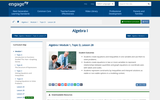Conditional Remix & Share Permitted
CC BY-NC-SA
Rating
0.0 stars
Overview:

Students create equations and inequalities in one variable and use them to solve problems.
Students create equations in two or more variables to represent relationships between quantities and graph equations on coordinate axes with labels and scales.
Students represent constraints by inequalities and interpret solutions as viable or non-viable options in a modeling context.

Subject:
Math 1
Material Type:
Lesson
Author:
EngageNY
02/03/2020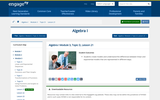Conditional Remix & Share Permitted
CC BY-NC-SA
Rating
0.0 stars
Overview:

Students create models and understand the differences between linear and exponential models that are represented in different ways.

Subject:
Math 1
Material Type:
Lesson
Author:
EngageNY
02/09/2020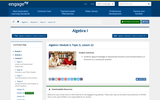Conditional Remix & Share Permitted
CC BY-NC-SA
Rating
0.0 stars
Overview:

Students apply knowledge of exponential functions and transformations of functions to a contextual situation.

Subject:
Math 1
Material Type:
Lesson
Author:
EngageNY
02/09/2020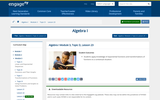Conditional Remix & Share Permitted
CC BY-NC-SA
Rating
0.0 stars
Overview:

Students apply knowledge of exponential functions and transformations of functions to a contextual situation.

Subject:
Math 1
Material Type:
Lesson
Author:
EngageNY
02/09/2020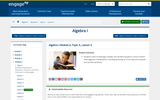Conditional Remix & Share Permitted
CC BY-NC-SA
Rating
0.0 stars
Overview:

Students solve increasingly complex one-variable equations, some of which need algebraic manipulation, including factoring as a first step and using the zero product property.

Subject:
Math 1
Material Type:
Lesson
Author:
EngageNY
02/09/2020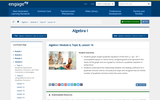Conditional Remix & Share Permitted
CC BY-NC-SA
Rating
0.0 stars
Overview:

Students graph simple quadratic equations of the form y = a(x - h)2 + k (completed-square or vertex form), recognizing that (h,k) represents the vertex of the graph and use a graph to construct a quadratic equation in vertex form.
Students understand the relationship between the leading coefficient of a quadratic function and its concavity and slope and recognize that an infinite number of quadratic functions share the same vertex.

Subject:
Math 1
Material Type:
Lesson
Author:
EngageNY
02/09/2020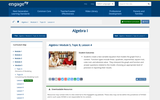Conditional Remix & Share Permitted
CC BY-NC-SA
Rating
0.0 stars
Overview:

Students create a two-variable equation that models the graph from a context. Function types include linear, quadratic, exponential, square root, cube root, and absolute value. They interpret the graph and function and answer questions related to the model, choosing an appropriate level of precision in reporting their results.

Subject:
Math 1
Material Type:
Lesson
Author:
EngageNY
02/09/2020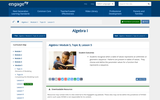Conditional Remix & Share Permitted
CC BY-NC-SA
Rating
0.0 stars
Overview:

Students recognize when a table of values represents an arithmetic or geometric sequence. Patterns are present in tables of values. They choose and define the parameter values for a function that represents a sequence.

Subject:
Math 1
Material Type:
Lesson
Author:
EngageNY
02/09/2020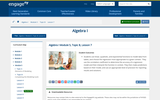Conditional Remix & Share Permitted
CC BY-NC-SA
Rating
0.0 stars
Overview:

Students use linear, quadratic, and exponential functions to model data from tables, and choose the regression most appropriate to a given context. They use the correlation coefficient to determine the accuracy of a regression model and then interpret the function in context. They then make predictions based on their model, and use an appropriate level of precision for reporting results and solutions.

Subject:
Math 1
Material Type:
Lesson
Author:
EngageNY
02/09/2020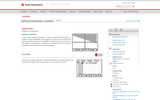Rating
0.0 stars
Overview:

Students will graph one linear inequality and then graph a system of linear inequalities as they apply to an area of land. Students will practice graphing systems of inequalities and determining if a points lies in the solution. In the extension, students are challenged to write a system of inequalities that describe the area of Mississippi.

Subject:
Mathematics
Material Type:
Activity/Lab
Provider:
Texas Instruments
03/07/2018Conditional Remix & Share Permitted
CC BY-NC-SA
Rating
0.0 stars
Overview:

CK-12 Foundation's Algebra FlexBook is an introduction to algebraic concepts for the high school student. Topics include: Equations & Functions, Real Numbers, Equations of Lines, Solving Systems of Equations & Quadratic Equations.

Subject:
Mathematics
Material Type:
Textbook
Provider:
CK-12 Foundation
Provider Set:
CK-12 FlexBook
12/06/2018Conditional Remix & Share Permitted
CC BY-NC-SA
Rating
0.0 stars
Overview:

This task was developed by high school and postsecondary mathematics and health sciences educators, and validated by content experts in the Common Core State Standards in mathematics and the National Career Clusters Knowledge & Skills Statements. It was developed with the purpose of demonstrating how the Common Core and CTE Knowledge & Skills Statements can be integrated into classroom learning - and to provide classroom teachers with a truly authentic task for either mathematics or CTE courses.

Subject:
Health Science Education
Material Type:
Activity/Lab
Lesson Plan
Provider:
National Association of State Directors of Career Technical Education Consortium
Provider Set:
Career Technical Education
07/31/2019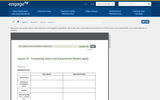Rating
0.0 stars
Overview:

Students create models and understand the differences between linear and exponential models that are represented in different way.

Subject:
Mathematics
Material Type:
Lesson Plan
Provider:
EngageNY
02/02/2018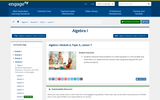Rating
0.0 stars
Overview:

Students will be able to interpret word problems to create equations in one variable and solve them (i.e., determine the solution set) using factoring and the zero product property

Subject:
Mathematics
Material Type:
Lesson Plan
Provider:
EngageNY
02/02/2018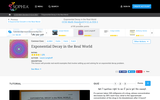Rating
0.0 stars
Overview:

This lesson will provide real world examples that involve setting up and solving for an exponential decay problem.

Subject:
Mathematics
Material Type:
Demonstration
Provider:
Sophia
04/17/2018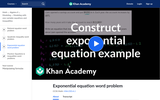Rating
0.0 stars
Overview:

This video models a context that concerns a bank savings account. The model turns out to be an exponential equation.

Subject:
Mathematics
Material Type:
Demonstration
Provider: